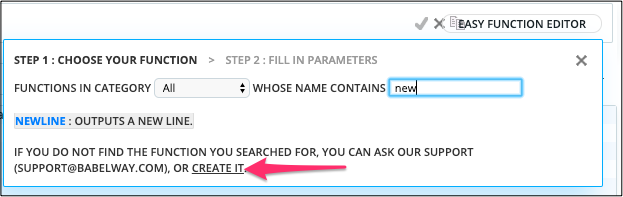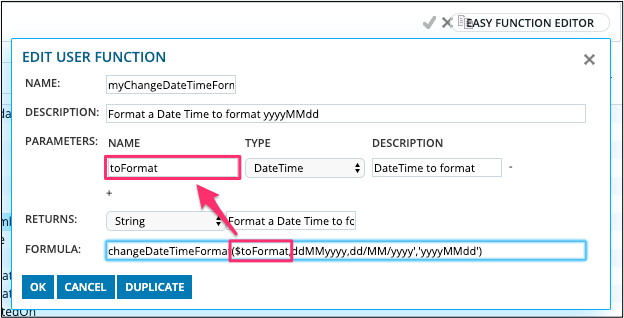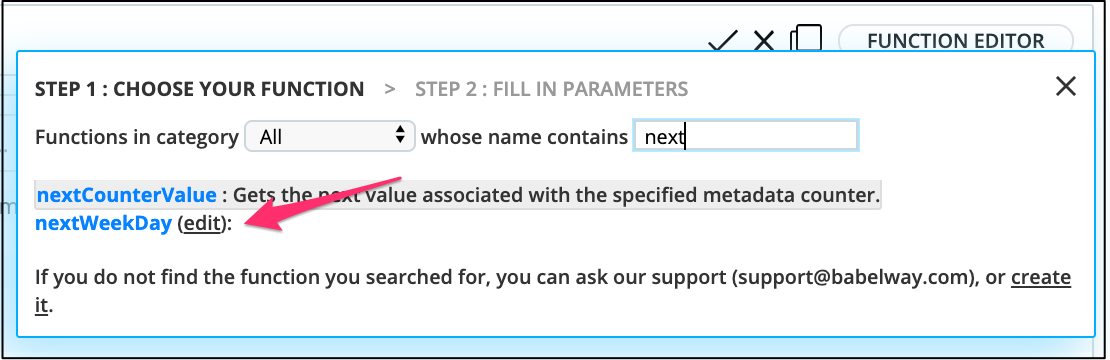Babelway offers many functions to make the calculations in your mappings. These functions should be enough for most of your needs. Anyway, in some situations, you could want to define additional functions, to simplify your mappings.

Specialize existing function to specific needs

The first reason why you could want to create additional functions is to specialize existing functions to your context.

As an example, let's suppose that you must convert in your mapping many dates, and that the input format is always ddMMyyyy or dd/MM/yyyy, and the output format must always be yyyyMMdd. In Babelway, this can be achieved easily by using the function changeDateTimeFormat.

changeDateTimeFormat([yourField], 'ddMMyyyy,dd/MM/yyyy', 'yyyyMMdd')

Anyway, having to type the input and output formats in every of these formulas can be tedious, and it would be easier to be able to write

convertDate([yourField])

This can be achieved by defining a function that suits this specific need :

convertDate(x) = changeDateTimeFormat(x, 'ddMMyyyy,dd/MM/yyyy', 'yyyyMMdd')

This scenario of specializing a method can be used in many situations : accessing a lookup table, formatting fields, ...

stock(x) = lookupTableValue('stocks', 'productId', 'stock', x)

Note: When using the changeDateTimeFormat() function with "Message Out" of type "Excel" then you will need to update the Excel template in the "Message Out" regarding the field that will have the output value of the changeDateTimeFormat() function to have the format cell of type "Text" in order to make sure that the value is generated as requested in the output message, as shown below.

Simplify complex formulas

Another situation where user functions are useful is when you have to write very complex (or long) formulas. Defining functions can simplify greatly the writing by allowing to factorize (and name) parts of the formulas.

In the following example, defining the intermediate function nextWeekDay has greatly improved the readability of the formula.

[DeliveryDate] = formatDateTime(nextWeekDay(nextWeekDay(currentDateTime())), 'yyyyMMdd')
nextWeekDay(day) = addDays(\$day, IF(formatDateTime(\$day, 'E')='Fri', 3, IF(formatDateTime(\$day, 'E')='Sat', 2, 1)))

The improvement in readability will increase with the complexity of the formula you have to write.

Definition in the interface

You have the ability to create your function from the screen with search of functions, in the function library.Defining user function (1)Defining user function (2)

All the concepts are the same as the one displayed for the already existing functions. See the section about standard functions for an in-depth explanation of every field. The fields are :

1. The function name identifies the function. It is what you have to write before the opening parenthesis to call this function.
2. The description describes what the function does, and what it is intended for. This description is not required for user functions, but filling it will allow the interface to show you help when you use the function, as for all the other functions.
3. The parameters section asks you for all the information about the parameters of the function.
1. The name of the parameter. It is just one word that should make you understand the parameter. It is also with this name that you will be able to reference the parameter in the implementation of the function.
2. Type of the parameter.
3. Description of the parameter.

4. The result section asks you for the type of the result of the function (a), and a small description of this result (b).
5. The formula is the formula that implements the function. In this formula, you can reference the function parameters with the dollar sign ('\$') followed by the name of the parameter.

Updating or deleting user functions

Starting again from the screen with list of functions, user functions have one more link to be able to edit them.

If you click on it, you will come again in the screen of the definition of the function, and you will be able to change the function, or delete it.Defining user function (2)

﻿﻿